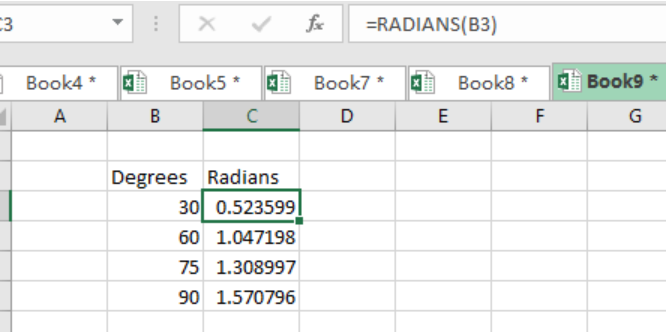Get instant live expert help with Excel or Google Sheets“My Excelchat expert helped me in less than 20 minutes, saving me what would have been 5 hours of work!”

#### Post your problem and you’ll get Expert help in seconds.

Your message must be at least 40 characters
Our professional Expert are available now. Your privacy is guaranteed.

# Does the Excel SIN Function Return Radians or Degrees?

• The question of whether the Excel function returns radians or degrees is common, with many people not being sure of the exact answer. To get an elaborate answer to this question, you will have to read and understand this post as it uses illustrations to bring out the answer more vividly.
• When we want to find the sine of an angle in excel, we are required to use the SIN function. The values of the angles are usually in degrees, and the SIN function will need the values to be converted before being supplied to it.
• To convert the values from degrees to radians, the RADIANS function in excel.
• Passing an angle in the form of degrees directly to the SIN function will result into an error, as the SIN function does not recognize the degrees.
• As already mentioned, we can use the Excel built-in function, the RADIANS, to convert the degrees into radians.

## Example

Let us convert 300  to radians. This can be as shown below

`=RADIANS(300)= 0.52`Figure 1. Final result

We can also use a mathematical formula to convert values from degrees to radians. This is as shown in the following illustration;

`Radians = Degrees* PI()/180`

The PI() is also a built-in function in excel, meaning you can use it whle working with excel worksheets.

## Instant Connection to an Expert through our Excelchat Service

Most of the time, the problem you will need to solve will be more complex than a simple application of a formula or function. If you want to save hours of research and frustration, try our live Excelchat service! Our Excel Experts are available 24/7 to answer any Excel question you may have. We guarantee a connection within 30 seconds and a customized solution within 20 minutes.

Solution examplesColumn A "Date Received" Column C "Priority" is validation list with options P1-P6 Column D "Due Date" .. How do I make Column D calculate the due date, based on which option is selected in Column C in conjunction with the date in Column A? PI = Date Received P2 = Date Received P3 = Date Received + 1 day P4 = Date Received + 5 business days P5 = Date Received + 14 business days P6 = Date Received + 30 business days
Solved by D. U. in 15 minsI am working in excel version 15.41 I want to compare to two large lists of doctors to determine matches based on NPI numbers. List are on 2 separate sheets (sheet 1 Column G and Sheet 2 Column A). I would like the sheet with duplications to be shown on Sheet 1 by highlighting the matching NPI numbers and lines that match. However I can not load the list because the file exceeds 20MB
Solved by E. L. in 60 minsHow can I create PIVOT Table for a large data having merged cell and free spaces, please
Solved by Z. L. in 29 minsIf I merged a multi-row, multi-column selection. Iand types some text into the merged cell and then unmerges it, what will happen to the text?
Solved by S. Y. in 60 minsIF SUM OF RANGE IS MORE THAN ZERO,THEN SUM IF THE RANGE IS BLANK THEN BLANK IF THE RANGE HAS ONLY ZERO,THEN ZERO HOW SHALL I COMBINE THE ABOVE THREE IN A SINGLE CELL??
Solved by M. D. in 18 mins